# Projective scheme

(diff) ← Older revision | Latest revision (diff) | Newer revision → (diff)

A closed subscheme (cf. Scheme) of a projective space. In homogeneous coordinates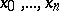on, a projective scheme is given by a system of homogeneous algebraic equations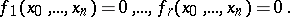Every projective scheme is complete (compact in the case); conversely, a complete scheme is projective if there is an ample invertible sheaf (cf. Ample sheaf; Invertible sheaf) on it. There are also other criteria of projectivity.

A generalization of the concept of a projective scheme is a projective morphism. A morphismof schemes is called projective (andis called a scheme projective over) ifis a closed subscheme of the projective fibre bundle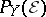, where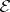is a locally free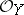-module. A composite of projective morphisms is projective. The projectivity of a morphism is preserved also under a base change; in particular, the fibres of a projective morphism are projective schemes (but not conversely). If a schemeis projective andis a finite surjective morphism, thenis also projective.

Any projective scheme (over) can be obtained using the construction of the projective spectrum (cf. Projective spectrum of a ring). Restricting to the case of an affine base,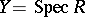, suppose thatis a graded-algebra with the-modulebeing of finite type and generating the algebra, and suppose thatis the set of homogeneous prime ideals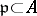not containing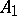. Equipped with the natural topology and a structure sheaf, the set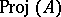is a projective-scheme; moreover, any projective-scheme has such a form.

How to Cite This Entry:
Projective scheme. Encyclopedia of Mathematics. URL: http://encyclopediaofmath.org/index.php?title=Projective_scheme&oldid=13162
This article was adapted from an original article by V.I. Danilov (originator), which appeared in Encyclopedia of Mathematics - ISBN 1402006098. See original article